﻿ Heat Diffusion in an Anisotropic Medium with Central Heat Source

### Heat Diffusion in an Anisotropic Medium with Central Heat SourceOPEN ACCESSPEER-REVIEWED

## Heat Diffusion in an Anisotropic Medium with Central Heat Source

Mohammad Sameti1, Alibakhsh Kasaeian1,1Department of Renewable Energies, Faculty of New Sciences and Technologies, University of Tehran, Tehran, Iran

### Abstract

Anisotropy is a property of some materials in which the physical properties vary with different crystallographic orientations. In this study, the analytical steady state solution to the anisotropic heat conduction in an orthotropic sphere is presented. There is a point heat source in the center of the sphere which supply the constant thermal energy. Using a transformation can change the anisotropic problem to an isotropic one which can be solved by the change of variables. The results showed that the isothermal surfaces are concentric ellipsoids which approach spheres while the conductivities in different directions are close to each other. The analytical results were validated with the 2D experimental tests performed for quartz as an orthotropic material. Despite the isotropic materials, it can be seen that the vectors of temperature gradients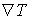and the heat flux q are not parallel.

### At a glance: Figures

12
Prev Next

• Sameti, Mohammad, and Alibakhsh Kasaeian. "Heat Diffusion in an Anisotropic Medium with Central Heat Source." International Journal of Partial Differential Equations and Applications 2.2 (2014): 23-26.
• Sameti, M. , & Kasaeian, A. (2014). Heat Diffusion in an Anisotropic Medium with Central Heat Source. International Journal of Partial Differential Equations and Applications, 2(2), 23-26.
• Sameti, Mohammad, and Alibakhsh Kasaeian. "Heat Diffusion in an Anisotropic Medium with Central Heat Source." International Journal of Partial Differential Equations and Applications 2, no. 2 (2014): 23-26.

 Import into BibTeX Import into EndNote Import into RefMan Import into RefWorks

### 1. Introduction

In heat transfer engineering, the heat flux law is usually considered for isotropic materials in which the thermal conductivity is independent from direction . There are many natural and synthetic materials in which the thermal conductivity varies with orientation and are called anisotropic materials. For example, crystals, wood, sedimentary rocks, metals that have undergone heavy cold pressing, laminated sheets, cables, heat shielding materials for space vehicles, fiber reinforced composite structures and many others are anisotropic materials . Instead, there are situations where anisotropic materials offer the only solution for the engineering requirements. As figure 1 illustrates, the heat shield for NASA's Mars Science Laboratory, is a good example for such applications .

Hsieh and Ma analyzed the steady state temperature and the heat flux fields in a thin-layer medium with anisotropic properties subjected to concentrated embedded heat sources or prescribed temperature on the surface. They used a linear coordinate transformation to transform anisotropic thinlayer problems into equivalent isotropic problems without complicating the geometry and boundary conditions of the problem . Shiah and Hwang applied the direct domain-mapping technique in the boundary element method (BEM) to investigate the heat conduction in composites consisting of multiple anisotropic media with embedded point heat sources . Li and Lai analyzed the influence of anisotropy of anisotropic soil on the processes of heat transfer by ground heat exchangers used in ground-coupled heat pump systems .

Figure 1. Large Heat Shield for Mars Science. It has a diameter of 4.5 meters and is the largest ever built for descending through the atmosphere of any planet 

In this study, an orthotropic sphere with radius R is considered in rectangular coordinates system. The orthotropic sphere has three mutually orthogonal axes so that its mechanical properties vary along each one. A point heat source generates thermal energy with the rate G at the center of the sphere and the temperature far from the sphere is T0. Alinear coordinate transformation is used to transform anisotropic 3D problem into the equivalent isotropic problem. The equivalent problem requires solving the Laplace equation with the prescribed boundary condition which is performed by the method of the separation of variables.

### 2. Formulation of the Problem

The general heat conduction equation in isotropic medium is the Fourier law as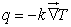(1)

where q is the heat flux vector, k is the thermal conductivity and T is the temperature. However, the heat conduction in the anisotropic material does not obey the Fourier law. The del operator has the following formula in an orthogonal coordinate (u1,u2,u3) system .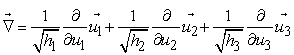(2)

Where hi is the metric factor and can be found by(3)

In the case of anisotropic materials, the heat flux can be compactly written as follows .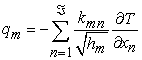(4)

In equation (4), x1=, x2= and x3=. Therefore, the heat flux is not essentially parallel to the temperature gradient [2, 8]. Figure 2 depicts how the heat diffuse in an anisotropic way through an anisotropic material. The heat equation is a result of energy balance equation :(5)

where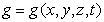is the heat generation, ρ is the density and Cp is the specific heat. By introducing equations (2) into equation (5) and assuming an orthotropic medium in which kmn=0 for mn and kmn=km for m=n, the following equation is obtained: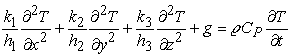(6)

The relations between new coordinates (u,v,w) and the Cartesian coordinates systems are assumed to be: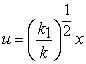(7)(8)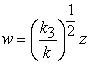(9)

and the metric factors can be readily found as:(10)

Where k is a reference conductivity.The choice of the reference conductivity is not arbitrary. The reason for this is that a volume element in the original space dxdydz transforms using equations (7) to (9) into equation (6) as: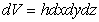(11)

where h=h1h2h3 is the metric factors product.

If the quantities ρCp and the generation term g defined on the basis of unit volume should have the same physical significance, so it is concluded that: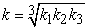(12)

Except for the center, there is no heat generation in the medium, so g=0. On the other hand, the problem was assumed to be steady, so the time derivative in equation (4) is equal to zero. Finally, equation (6) is simplified to the well-known Laplace equation: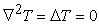(13)

or(14)

One way for the equation to be solved, is using the method of separation of variables . Instead, the problem is solved in another way, here. Because the geometry, the problem and the boundary conditions are symmetric, it can be assumed that changing any of the variables with another one, would not change the final result. So, the solution can be considered as a function of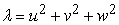which is the simplest assumed function, because it is polynomial and has the minimum power to be differentiated in equation (14). Using the chain rule, the first term in equation (14) is transformed into: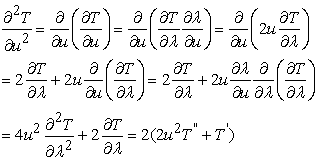(15)

where prime and double prime denotes the first and the second derivatives, respectively. The same expression can be found for other terms and the equation (14) is simplified to: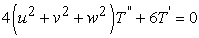(16)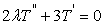(17)

which simply can be solved by considering τ=T and finding the solution of the first order differential equation by means of separation. As a result, the solution for temperature distribution can be expressed as:(18)

The infinity boundary condition should be satisfied, which is:(19)

which means that B=T0. Assuming a very small sphere with radius ε around the center of the system, it is concluded that the heat generation G should be conducted through the small sphere which is known as surface energy balance. Mathematically, it can be written as:(20)

or(21)

and the final solution for T(x,y,z) can be achieved by: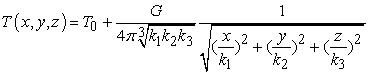(22)

### 3. Results and Discussions

It can be seen from equation (22) that the isothermal surfaces are ellipsoids with the equation: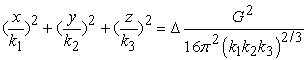(23)
Figure 3. Heat flow patterns on the sensitive film. The thermal conductivity are equal in the a- and b-directions but differs from its value in the c-direction. The above figure is a circle while the below one is an ellipse 

Where Δ is the temperature difference of the isothermal surface with the center? A simple test has been done in University of Cambridge which verifies the solution for the isothermal surfaces. When heating the section of a material made from quartz, two different observations can be recorded. When the section is cut perpendicularly to the one axis, the observed shape is a circle, showing that the thermal conductivity is the same in all directions in this plane. However, when using the section cut parallel to that axes, the shape seen is an ellipse, which shows that the thermal conductivity in this plane is direction-dependent . The heat flow patterns for the above situations are illustrated in figure 3.

It is also obvious that the Δ=T-T0 decreases with decreasing distance from the origin. It can be seen from equation (22) that the heat flux vector is parallel to the position vector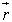but the isothermal surface is normal to the temperature gradient vector. So, it is concluded that despite the isotropic heat transfer, the heat flux and isothermal surfaces are not parallel. Figure 4 depicts these vectors.

Figure 4. Ellipsoid isothermal surfaces, the heat flow vector and the temperature gradient vector

### 4. Conclusion

Heat conduction in anisotropic materials has numerous important applications in various branches of science and technologies. An orthotropic sphere is considered in rectangular coordinates system. A point heat source generates thermal energy with constant rate at the origin of the sphere and the temperature far from the sphere is uniform. A linear coordinate transformation is used to transform anisotropic 3D problem into the equivalent isotropic one without complicating the geometry and boundary conditions of the problem. The isotropic problem is solved by means of change of variables. It is obtained that the isothermal surfaces are ellipsoids and the heat flux vectors are not parallel to temperature gradient vector. If the problem was isotropic, these vectors would be parallel. The analytical results were validated with the 2D experimental tests performed for quartz as an orthotropic material.

### References

  Çengel, Y. A., & Ghajar, A. J. (2014). Heat and mass transfer: fundamentals & applications. McGraw-Hill.In article  Sameti, M., Astaraie, F. R., Pourfayaz, F., & Kasaeian, A. (2014). Analytical and FDM Solutions for Anisotropic Heat Conduction in an Orthotropic Rectangular. American Journal of Numerical Analysis, 2 (2), 65-68.In article  www.nasa.gov/mission_pages/msl/msl-20090710.html (accessed 2014/24/03).In article  Hsieh, M. H., & Ma, C. C. (2002). Analytical investigations for heat conduction problems in anisotropic thin-layer media with embedded heat sources.International journal of heat and mass transfer, 45 (20), 4117-4132.In article CrossRef  Shiah, Y. C., Hwang, P. W., & Yang, R. B. (2006). Heat conduction in multiply adjoined anisotropic media with embedded point heat sources. Journal of heat transfer, 128 (2), 207-214.In article CrossRef  Li, M., & Lai, A. C. (2012). Heat-source solutions to heat conduction in anisotropic media with application to pile and borehole ground heat exchangers. Applied Energy, 96, 451-458.In article CrossRef  Hayek, S. I. (2000). Advanced mathematical methods in science and engineering. CRC Press.In article  Ozisik, M. N. (2013). Boundary value problems of heat conduction. Courier Dover Publications.In article  Boyce, W. E., & DiPrima, R. C. (2012). Elementary differential equations and boundary value problems.In article  www.doitpoms.ac.uk/tlplib/anisotropy/thermal.php (accessed 2014/25/03)In article